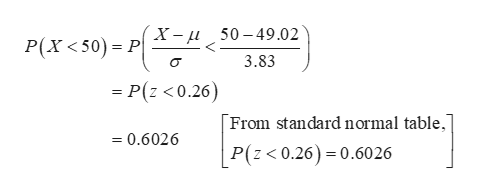# Suppose that the weights of airline passenger bags are normally distributed with a mean of 49.02 pounds and a standard deviation of 3.83 pounds.a) What is the probability that the weight of a bag will be less than the maximum allowable weight of 50 pounds? Give your answer to four decimal places.b) Let X represent the weight of a randomly selected bag. For what value of c is P(E(X) - c < X < E(X) + c)=0.82? Give your answer to four decimal places.c) Assume the weights of individual bags are independent. What is the expected number of bags out of a sample of 17 that weigh less than 50 lbs? Give your answer to four decimal places.d) Assuming the weights of individual bags are independent, what is the probability that 11 or fewer bags weigh less than 50 pounds in a sample of size 17? Give your answer to four decimal places.

Question

Suppose that the weights of airline passenger bags are normally distributed with a mean of 49.02 pounds and a standard deviation of 3.83 pounds.

a) What is the probability that the weight of a bag will be less than the maximum allowable weight of 50 pounds? Give your answer to four decimal places.

b) Let X represent the weight of a randomly selected bag. For what value of c is P(E(X) - c < X < E(X) + c)=0.82? Give your answer to four decimal places.

c) Assume the weights of individual bags are independent. What is the expected number of bags out of a sample of 17 that weigh less than 50 lbs? Give your answer to four decimal places.

d) Assuming the weights of individual bags are independent, what is the probability that 11 or fewer bags weigh less than 50 pounds in a sample of size 17? Give your answer to four decimal places.

check_circle

Step 1

Hello! As you have posted 4 sub parts, we are answering the first 3 sub-parts.  In case you require the unanswered part (d) also, kindly re-post that part separately.

a)

The probability that the weight of a bag will be less than the maximum allowable weight of 50 pounds is 0.6026 and it is obtained below:

From the given information, let us define the random variable X as the weights of airline passenger bags are normally distributed with mean of 49.02 and standard deviation of 3.83.help_outlineImage TranscriptioncloseP(X<50) = PX= i_50-49.02 3.83 = P(z <0.26) From standard normal table," =0.6026 P(z< 0.26) 0.6026 fullscreen
Step 2

b)

The value of c is 5.1705 and it is com...

### Want to see the full answer?

See Solution

#### Want to see this answer and more?

Solutions are written by subject experts who are available 24/7. Questions are typically answered within 1 hour.*

See Solution
*Response times may vary by subject and question.
Tagged in

### Other